GRE Subject Test: Chemistry : Acid-Base Analysis

Example Questions

← Previous 1

Example Question #1 : Titration Curves

Consider the titration curve, in which an acid is treated with aqueous 0.5M NaOH.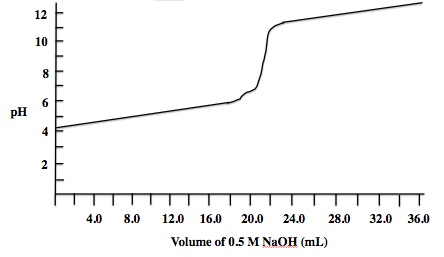The pH at the equivalence point was found to be 8.9. Approximately how many milliliters of 0.5M NaOH were needed to reach equivalence?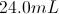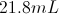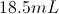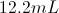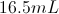Explanation:

For any titration curve the equivalence point corresponds to the steepest part of the curve. In this case, the question indicates that the pH at equivalence was 8.9, which would correspond to a volume between 20.0 and 24.0 on the x-axis of the graph. This leads to our approximate answer of 21.8mL.

Example Question #8 : Titration Curves

Consider the titration curve, in which an acid is treated with aqueous 0.5M NaOH.What is the approximate pKa of the starting acid?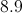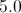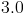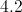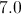Explanation:

The pKa corresponds to the pH when half of the acid has been neutralized. In this case, that would correspond to when half of the 0.5M NaOH has been added. We can see in the curve that the equivalence point is at around 22mL of NaOH, as this is the steepest change in pH. The half equivalence point will be found at approximately 11mL of NaOH addition. On the graph, 11mL of NaOH results in a pH of approximately 5.0, giving us our pKa value.

Example Question #1 : Titration Curves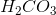is a diprotic acid. Which of the following statements is true about its second half equivalence point?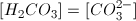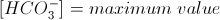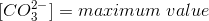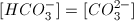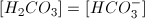Explanation:

H2CO3 will have two equivalence points and two half equivalence points, one set corresponding to each of its two protons.

After the first equivalence point, all of the acid is in the form HCO3-. When an acid is at a half equivalence point, the acid's concentration will be equal to the concentration of the conjugate base. For the second half equivalence point, the acid is in the  HCO3- form, and the conjugate base is CO32-. As a result, at the second half equivalence point, the concentrations of HCO3- and CO32- will be equal.

Example Question #2 : Analyzing Reactions

NaOH is added to a 500mL of 2M acetic acid. If the pKa value of acetic acid is approximately 4.8, what volume of 2M NaOH must be added so that the pH of the solution is 4.8?

250mL

2L

500mL

1L

250mL

Explanation:

To solve this question you need to think about the chemical reaction occurring.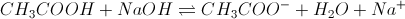We can ignore water and sodium ions for the sake of this question. The reactants exist in a 1:1 ratio, so that for every mol of NaOH we add, we lose one mol of acetic acid and gain one mol of acetate. We can determine the moles of acetic acid by using M = mol/L, which gives us mol = ML = (2M) * (0.5L) = 1mol acetic acid. If we use the Hendersen Hasselbach equation we can see that the pH equals the pKa when the concentration of conjugate base (acetate) equals the concentration of acid.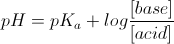If we have 1mol of acetic acid and add 0.5mol of NaOH, we will lose 0.5mol of acetic acid and gain 0.5mol of acetate. We will then be at a point where acetic acid equals acetate. This is summarized in the ICE table below. Now we know the moles of NaOH (0.5 moles) and the concentration (2M) so we can find the volume by doing M = mol/L.

L = mol/M = (0.5mol)/(2M) = 0.25L

 Acetic acid NaOH Acetate I 1 mol 0.5 mol 0 mol C -0.5 mol -0.5 mol +0.5 mol E 0.5 mol 0 mol 0.5 mol

Example Question #1 : Henderson Hasselbalch Equation

The Ka for HCN is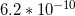.

If there is a solution of 2M HCN, what concentration of NaCN is needed in order for the pH to be 9.2?

1M

No NaCN needs to be added

3M

2M

2M

Explanation:

To answer this question, we need to be able to compare the concentrations of acid and conjugate base in the solution with the pH. The Henderson-Hasselbach equation is used to compare these values, and is written as: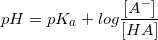. In this question, this equation can be written with the given values of Ka and pH.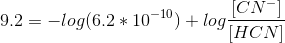Since we know the Ka of HCN, we can derive the pKa, which turns out to be 9.2.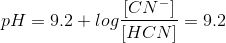As a result, we want to see to it that the amount of conjugate base is equal to the concentration of acid, so that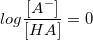. Because log(1) = 0, we want to see to it that the concentrations of the acid and the conjugate base are equal to one another. We know from the question that [HCN] = 2M.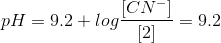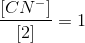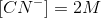As a result, a concentration of 2M NaCN will allow the pH of the solution to be 9.2.

Example Question #1 : Henderson Hasselbalch Equation

Calculate the concentration of hydrogen ions in the following acetic acid solution.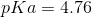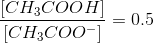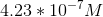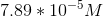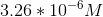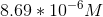Explanation:

To answer this question you need to use the Henderson-Hasselbalch equation: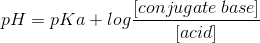The ratio given in the question is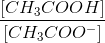, or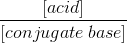.

To use the correct ratio for the Henderson-Hasselbalch equation, we need to convert this ratio to its reciprocal: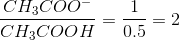Plugging the given values into the equation gives us: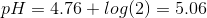The question is asking for the concentration of hydrogen ions. To solve for this we have to use the definition of pH.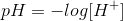Solving for the concentration of hydrogen ions gives us: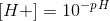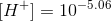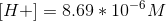Example Question #7 : Henderson Hasselbalch Equation

Which of the following is true regarding the Henderson-Hasselbalch equation?

I. The pH of the solution is always greater than the pKa of the solution

II. As the ratio of conjugate base to acid increases, the pH increases

III. The hydrogen ion concentration can never equal the acid dissociation constant

I only

II and III

I and II

II only

II only

Explanation:

The Henderson-Hasselbalch equation is a tool that allows us to calculate the pH of an acid solution using the pKa of the acid and the relative concentrations of the acid and its conjugate base. It is defined as: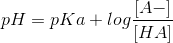By looking at the equation we can determine that if the ratio inside the logarithm is greater than 1, then the pH of the solution will be greater than the pKa; however, if the ratio is less than 1 (meaning, if the concentration of the acid is greater than the concentration of conjugate base), then the pH will be less than the pKa. Statement I is false.

Increasing the ratio of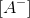to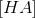will increase the logarithm, and subsequently the pH of the solution. This makes sense because you will have more conjugate base than acid, thereby making the solution more alkaline and increasing the pH. Statement II is true.

pH and pKa are defined as follows: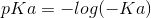If we have the same concentration of hydrogen ions as the acid dissociation constant (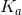), then the pH will equal the pKa. According to the Henderson-Hasselbalch equation, the pH equals the pKa if the concentration of the conjugate base equals the concentration of acid; therefore, it is possible for the hydrogen ion concentration to equal the acid dissociation constant. Statement III is false.

Example Question #1 : Henderson Hasselbalch Equation

A researcher prepares two solutions. Solution A contains an unknown acid, HA, and solution B contains an unknown acid, HB. The researcher performs several tests and collects the following data.

1. Both solutions contain weak acids

2.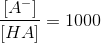3.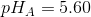4.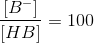5.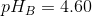What can you conclude about these two solutions?

The hydrogen ion concentration of solution A is greater than that of solution B

Acid HB is more acidic than acid HA

The acids, HA and HB, are identical

The acid dissociation constant for HA is greater than that of HB

The acids, HA and HB, are identical

Explanation:

The Henderson-Hasselbalch equation states that: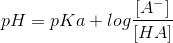The question gives us information regarding the ratio of conjugate base to acid AND the pH for each acidic solution. Using this information, we can solve for the pKa values of both solutions.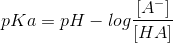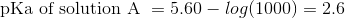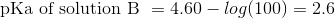The pKa values of both solutions are the same. This means that both solution contains the same acid; therefore, the identity of HA is the same as the identity of HB.

The hydrogen ion concentration of solution A is lower than that of solution B because the pH of solution A is greater. Acidity, or strength, of an acid is determined by the pKa. Since we have the same pKa for both acids, HA and HB will have the same acidity. Acid dissociation constant, Ka, is defined as: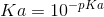Acid dissociation constant only depends on the pKa; therefore, the Ka for both acids is the same.

Example Question #1 : Acid Base Analysis

47.0g of nitrous acid, HNO2, is added to 4L of water. What is the resulting pH?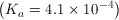2.5

3.0

2.0

3.5

3.2

2.0

Explanation:

HNO2 is a weak acid; it will not fully dissociate, so we need to use the HA → H+ A reaction, with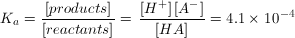.

47.0g HNO2 is equal to 1mol. 1mol into 4L gives a concentration of 0.25M when the acid is first dissolved; however, we want the pH at equilibrium, not at the initial state. As the acid dissolves, we know [HNO2] will decrease to become ions, but we don't know by how much so we indicate the decrease as "x". As HNO2 dissolves by a factor of x, the ion concentrations will increase by x.

HNO2 → H+ NO2

Initial             0.25M       0      0

Equilibrium     0.25 – x     x      x

Now, we can fill in our equation: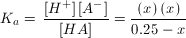.

Since x is very small, we can ignore it in the denominator: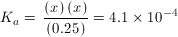(they expect you to do this on the MCAT; you will never have to solve with x in the denominator on the exam!)

Solve for x, and you find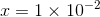. Looking at our table, we know that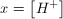Now we can solve for pH: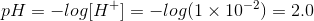Example Question #21 : Analytical Chemistry

What is the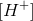of a 0.12M solution of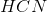that has a pH of 5.1?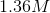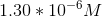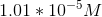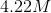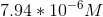Explanation:

We can calculate the hydrogen ion concentration by using the following equation: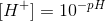Plug in the given value for pH and solve: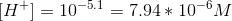← Previous 1

All GRE Subject Test: Chemistry Resources